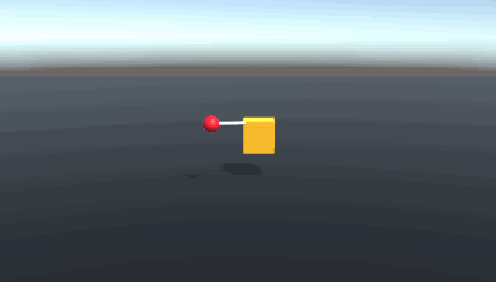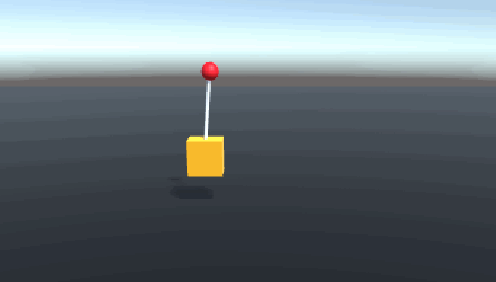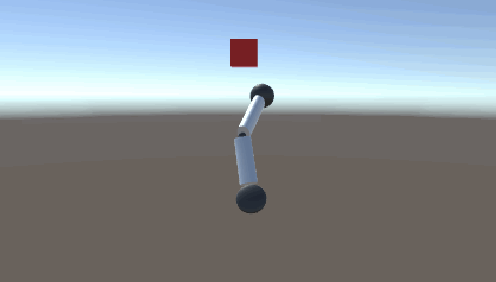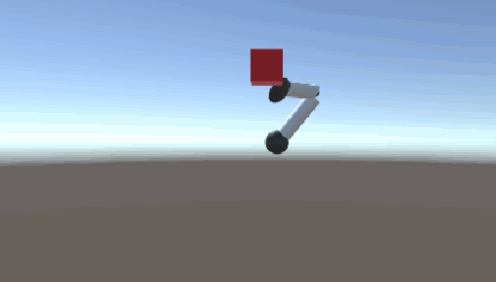Unity Physics Reinforcement Learning by RnkSngh - 1

Physics

Teaching robots physics without teaching robots physics

Unknown VersionUnknown LicenseUpdated 155 days agoCreated on July 28th, 2020
Go to source

Unity-Physics-Reinforcement-Learning

This folder contains two separate Unity projects - a 3D inverted pendulum, and a robot arm, that explore using Unity as a physics engine to simulate reinforcement learning agents. Instead of using closed form solutions to accomplish their respective tasks - the agents use some arbitrary mapping of observations to actions that was learned through training. Both agents use proximal policy optimization (as the reward was given at each timestep and was thus not sparse), a discount factor gamma of 0.99. The state value function for both agents was approximated using a neural net with 128 hidden units over 2 hidden layers.

Untrained 3D Inverted PendulumTrained 3D Inverted PendulumUntrained Robot ArmTrained Robot Arm3D Inverted Pendulum

The 3D inverted Pendulum problem is a generalization of the inverted pendulum problem. Instead of a rectangle constrained to move in a 1D line to balance the attached weight, the 3D inverted pendulum consists of a cube constrained to move in a 2D plane to balance a weight.

Reward Function

The height of the rod is maximized when the rod is perfectly balanced; thus the agent was trained to maximize the y position of the weight being balanced.

Input Observations

The neural net takes in a 10 dimensional input. 4 of the 10 inputs represent the x and z coordinates of the position and velocity of the actuated cube (y was neglected as the cube cannot move in the y direction). The other 6 inputs represent the x, y, and z positions and velocities of the weight being balanced. The positions and velocities of the weight were transformed to a local coordinate system before being passed on as inputs, as only the relative positions and velocities between the weight and the cube are relevant to balancing the weight.

Actions

The agent indirectly controls the x and z velocities of the cube by applying forces to move the cube within the xz plane. Thus, the agent gives a 2 dimensional output that represents the force, up to 25 Newtons, to be applied to the cube in the x and z directions. The position and velocity of the balanced weight was controlled indirectly by the connection between the cube and the rod and the connection between the rod and the weight.

Reset Conditions

Training episodes were reset if the y component of the weight was negative (i.e. if the weight fell below the cube) or if the cube’s position moved outside of a 10x10 square centered on the origin in the xz plane.

Robot Arm

The Robot Arm project contains an abstraction of an arm that is fixed at the shoulder. The agent solves the task of navigating the hand to a randomly generated goal position, shown by a red cube. The goal position is constrained to always spawn within the arm’s reach, which can be represented by a sphere with a radius equal to the arm’s length. The arm components are modeled as rigid bodies (i.e. no deformations were modeled) and thus all possible movements of the arm can be modeled as a set of independently applied torques to both joints.

This project was inspired by another Unity robot arm project, though the agent in this repo uses torque-based actuation to control the arm rather than directly controlling the angles of joints. A torque-based actuation was chosen to explore whether the agent could find the correct sequence of torque applications to overcome gravity to lift the arm up to positions.

Reward Function

The agent was trained to minimize the distance between the target cube and the hand (though reward functions are typically framed as a maximization problem, and thus was framed as maximizing the negative of the distance between the target cube and the hand).

Observations

The neural net takes in a 16 dimensional observation input. 12 of the 16 dimensions are the x, y, and z positions and velocities of the hand and elbow. Similar to the weight inputs for the cube agent, the elbow inputs use a local coordinate system to define relative positions and velocities with respect to the hand’s relative positions and velocities. 4 of the 16 dimensions represent a quaternion of the orientation of the shoulder joint.

Actions

The agent outputs a 4 dimensional output vector of torques to be applied to the joints. 1 output dimension indicates the torque to be applied to the elbow joint (which is modeled as a hinge joint and thus can only rotate about a single axis). 3 output dimensions indicate the torques to be applied about 3 axes on the shoulder joint (which is modeled as a ball and socket joint and thus can rotate about 3 axes).

Reset Conditions

Training episodes were reset once the arm reached the goal, which was found by checking if the hand was within a given tolerance distance (chosen to be 0.7 meters) within the center of the target cube.

Show all projects by RnkSngh